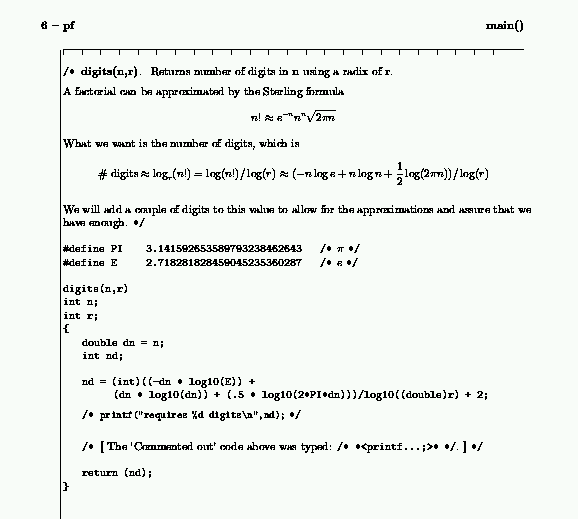IDENTITY AND ACCESS MANAGEMENT
• Updated: Apr 5, 1999

 Format c with tex

This is the cnoweb literate programming' demonstration program (pf.c) and macro package (cnoweb.tex) as described in TugBoat volume 11, number 4.

The package allows you to both compile (with cc) and format (with tex) the same source file.

For example, this code fragment from pf.c

 / * \subsection{\"digits(n,r)"} Returns number of digits in \"n" using a radix of \"r". A factorial can be approximated by the Sterling formula $$n! \approx e^{-n} n^{n} \sqrt{2\pi n}$$ What we want is the number of digits, which is $$\hbox{\# digits} \approx \log_r(n!)=\log(n!)/\log(r) \approx (-n \log e + n \log n + {1\over2}\log (2\pi n))/\log(r)$$ We will add a couple of digits to this value to allow for the approximations and assure that we have enough. * / digits(n,r) int n; int r; { double dn = n; int nd; nd = (int)((-dn * log10(2.718281828459045235360287 / * $e$ * /)) + (dn * log10(dn)) + (.5 * log10(2*3.141592653589793238462643 / * $\pi$ * /*dn)))/log10((double)r) + 2; / * ** * / / * \nweb The Commented out' code above was typed: {\tt/{}* *{}{}* *{}/}.\bewn * / return (nd); } 

produces this document.Jim Fox UW Technology Identity and Access Management University of Washington fox@washington.edu